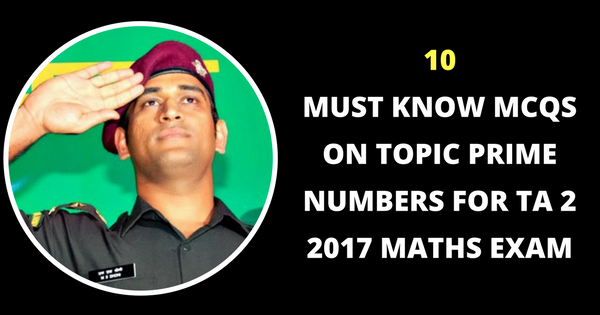# 10 Must Know MCQs On Topic Prime Numbers For TA 2 2017 Maths Exam

The question paper will be designed to test the candidates ability to complete sequences making logical conclusion based on simple patter of numbers, statements, figures, letters etc as may be expected of a rational thinking person without any special study of the subject.

# Territorial Army 2 2017 Exam Mock Test Series## 10 Must Know MCQs On Topic Prime Numbers For TA 2 2017 Maths Exam• A prime number has only two factors: 1 and itself.
• A composite number has more than two factors.
• The number 1 is neither prime nor composite.

1- Which one of the following is not a prime number?
 A. 31 B. 61 C. 71 D. 91

91 is divisible by 7. So, it is not a prime number.

2- Which of the following is a prime number ?
 A. 33 B. 81 C. 93 D. 97

Clearly, 97 is a prime number.

3- The sum of first five prime numbers is:
 A. 11 B. 18 C. 26 D. 28

Required sum = (2 + 3 + 5 + 7 + 11) = 28.

Note: 1 is not a prime number.

4-The smallest 3 digit prime number is:
 A. 101 B. 103 C. 109 D. 113

The smallest 3-digit number is 100, which is divisible by 2.

100 is not a prime number.

101 < 11 and 101 is not divisible by any of the prime numbers 2, 3, 5, 7, 11.

101 is a prime number.

Hence 101 is the smallest 3-digit prime number.

5- If the number 481 * 673 is completely divisible by 9, then the smallest whole number in place of * will be:
 A. 2 B. 5 C. 6 D. 7 E. None of these

Sum of digits = (4 + 8 + 1 + x + 6 + 7 + 3) = (29 + x), which must be divisible by 9.

x = 7.

6- On dividing 2272 as well as 875 by 3-digit number N, we get the same remainder. The sum of the digits of N is:
 A. 10 B. 11 C. 12 D. 13

Clearly, (2272 – 875) = 1397, is exactly divisible by N.

Now, 1397 = 11 x 127

The required 3-digit number is 127, the sum of whose digits is 10.

7- How many prime numbers are less than 50 ?
 A. 16 B. 15 C. 14 D. 18

Prime numbers less than 50 are:
2, 3, 5, 7, 11, 13, 17, 19, 23, 29, 31, 37, 41, 43, 47

Their number is 15

(xn – an) is completely divisible by (x – a), when
 A. n is any natural number B. n is an even natural number C. n is and odd natural number D. n is prime

For every natural number n, (xn – an) is completely divisible by (x – a).

Which one of the following is a prime number ?
 A. 161 B. 221 C. 373 D. 437 E. None of these

437 > 22

All prime numbers less than 22 are : 2, 3, 5, 7, 11, 13, 17, 19.

161 is divisible by 7, and 221 is divisible by 13.

373 is not divisible by any of the above prime numbers.

373 is prime.

Which one of the following can’t be the square of natural number ?
 A. 30976 B. 75625 C. 28561 D. 143642

The square of a natural number nerver ends in 2.

143642 is not the square of natural number.#### Ashutosh

Defence exams mentor, cracked CDS and AFCAT exams.

View all Posts